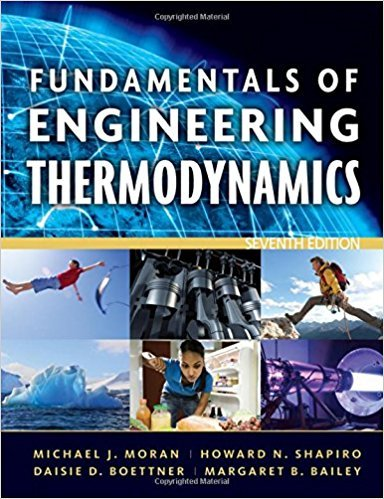×
×

# Evaluating Heat TransferA composite plane wall consists ofISBN: 9780470495902 50

## Solution for problem 47P Chapter 2

Fundamentals of Engineering Thermodynamics | 7th Edition

• Textbook Solutions
• 2901 Step-by-step solutions solved by professors and subject experts
• Get 24/7 help from StudySoup virtual teaching assistantsFundamentals of Engineering Thermodynamics | 7th Edition

4 5 0 261 Reviews
16
5
Problem 47P

Evaluating Heat Transfer

A composite plane wall consists of a 75-mm-thick layer of insulation (ki = 0.05 W/m · K) and a 25-mm-thick layer of siding (k = 0.10 W/m · K). The inner temperature of the insulation is 20°C. The outer temperature of the siding is —13°C. Determine at steady state (a) the temperature at the interface of the two layers, in °C, and (b) the rate of heat transfer through the wall, in W per m2 of surface area.

Step-by-Step Solution:
Step 1 of 3

MICROBIOLOGY [BIOL 2300] NOTES WEEK OF JAN 10 AND WEEK OF JAN 17 CHAPTER 1 HUMANS AND THE MICROBIAL WORLD OTHER IMPORTANT MED TERMS Normal flora is the human microbiome. Objective: data through test Another name for this is...

Step 2 of 3

Step 3 of 3

##### ISBN: 9780470495902

The answer to “Evaluating Heat TransferA composite plane wall consists of a 75-mm-thick layer of insulation (ki = 0.05 W/m · K) and a 25-mm-thick layer of siding (k = 0.10 W/m · K). The inner temperature of the insulation is 20°C. The outer temperature of the siding is —13°C. Determine at steady state (a) the temperature at the interface of the two layers, in °C, and (b) the rate of heat transfer through the wall, in W per m2 of surface area.” is broken down into a number of easy to follow steps, and 80 words. This full solution covers the following key subjects: temperature, siding, thick, Wall, transfer. This expansive textbook survival guide covers 14 chapters, and 1501 solutions. The full step-by-step solution to problem: 47P from chapter: 2 was answered by , our top Engineering and Tech solution expert on 07/20/17, 09:01AM. This textbook survival guide was created for the textbook: Fundamentals of Engineering Thermodynamics, edition: 7. Fundamentals of Engineering Thermodynamics was written by and is associated to the ISBN: 9780470495902. Since the solution to 47P from 2 chapter was answered, more than 292 students have viewed the full step-by-step answer.

Unlock Textbook Solution